## Introduction

Cavity quantum electrodynamics (QED) and its low-frequency on-chip counterpart circuit QED form prototypical quantum systems that have enabled many tests of quantum theory and advanced goals of quantum computation1,2. A single cavity QED (cQED) system links a quantum two-level system (qubit) to excitations of the electromagnetic field. Linking multiple, spatially separated cQED systems with electromagnetic waves provides a scalable quantum networking architecture that could combine a large number of individual systems (or nodes) into one network3,4,5. Deterministically distributing information between different nodes requires the electromagnetic signals generated by one node be completely absorbed by another. This requirement can be viewed as a generalized impedance matching condition, which can be satisfied by controlling the temporal and spectral content of the signal6,7.

The content of an electromagnetic signal can be controlled using a suitable nonlinearity. For example, at microwave frequencies, the nonlinear inductance provided by Josephson junctions has been used to shape the temporal envelope of signals8,9,10,11 or alter their frequency12,13,14. Apart from Josephson junctions, the nonlinear interaction between electricity (or light) and a vibrating mass has been used to store electromagnetic signals15,16,17 and convert them between different frequencies18,19,20. However, no scheme has yet demonstrated arbitrary temporal and spectral control of electromagnetic signals using a single device.

In this work, we combine microwave-frequency pulse shaping and conversion between adjustable frequencies by embedding a mechanical oscillator in a tunable circuit. As a demonstration of our temporal and spectral mode converter, we use it to implement a particular protocol that converts the temporal envelope of a 7 GHz microwave signal from a decaying exponential to a Gaussian, and also shifts the signal’s frequency by up to 250 MHz. Such a protocol can process signals emitted from coherent circuit QED systems, which emit signals in a fixed, narrow (10 MHz) frequency range, and with an exponentially decaying envelope21,22. To test the suitability of the mode converter for use with quantum signals, we characterize the noise acquired by low-amplitude classical signals during temporal and spectral mode conversion. We find the total added noise is less than one quantum for frequency shifts up to approximately 100 MHz, and 0.4 quanta for small frequency shifts, indicating that the mode converter approaches the performance required to preserve fragile quantum signals. (If the added noise is larger than half a quantum, negative features of a signal’s Wigner quasi-probability distribution vanish23.)

## Results

### Tunable electromechanical circuit

Vibrations of the drumhead are also affected by the electromechanical coupling G. At Vdc=0, G=2π × 42 MHz nm−1, and in the presence of a detuned microwave pump, this coupling exchanges excitations of the microwave circuit and vibrational excitations of the drumhead at a rate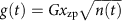(ref. 16), where xzp=6.4 fm is the zero-point motion of the mechanical oscillator and n(t) is the strength of the microwave pump expressed as the number of photons induced in the circuit by the pump. We choose to work in the weak-coupling regime, where excitations in the microwave circuit decay into the transmission line (see Fig. 1a) at a rate κext>2g. In this regime, the microwave circuit simply enhances an exchange between excitations in the transmission line and vibrational excitations of the drumhead25; this exchange occurs at a rate Γ(t)=4g(t)2/κ, where κ is the total energy decay rate of the microwave circuit. The controllable, time-dependent coupling to a transmission line provided by this device is a basic requirement for controlling the temporal envelope of signals7.

### Mode conversion protocol

Our protocol for mode conversion consists of modulating both Vdc and Γ(t). Initially, we modulate Γ(t) to capture a microwave signal propagating down the transmission line and store it as a vibration of the drumhead26. The signal can be stored as a vibration for over (nthκm)−1≈180 μs before it gains one quantum of energy from the environment. This storage time is sufficient to complete the mode conversion protocol, and can be adjusted to serve as a signal delay. Once the signal is captured, we change Vdc to alter the microwave circuit’s resonant frequency. We then modulate Γ(t) to release the signal stored in the drumhead back into the transmission line. Even though the released signal has different spectral and temporal content from the initial signal, the information in the initial signal has ideally been preserved. The protocol is schematically depicted in Fig. 2.

Signals reflected or emitted from the mode converter are measured with a microwave receiver that consists of a Josephson parametric amplifier27 followed by additional amplifiers, a down-converting mixer and a digitizer (Supplementary Note 2, Supplementary Fig. 3). Collected data consist of time-stamped voltage values that we coherently average by running multiple repetitions of the mode conversion protocol. To clearly represent the time and frequency content of a measured voltage waveform, we construct the Wigner–Ville distribution using the discretized version of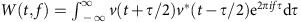, where W(t, f) is the Wigner–Ville distribution and v(t) is the analytic voltage measured at time t. The analytic voltage is constructed using a Hilbert transform on the measured voltage28: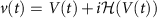, where V(t) is the voltage measured at time t and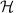is the Hilbert transform. The resulting time-frequency distribution is related to the position-momentum Wigner quasi-probability distribution; however, nothing ‘quantum’ is associated with a negative distribution. The marginal distributions of the Wigner–Ville distribution give the temporal envelope and energy spectral density of the voltage waveform.

### Mode conversion results

As an initial test of our measurement apparatus, we generate a signal near 7.08 GHz that has an exponentially decaying envelope (power decay rate of 2π × 24 kHz) and inject it into the coaxial transmission line that connects to the mode converter. We choose a signal with this temporal envelope because it resembles the temporal envelope of quantum signals emitted from highly coherent circuit QED systems21. We initially set Vdc so that the microwave circuit has a resonant frequency substantially different from the injected signal, and the signal reflects off the mode converter and is measured by our microwave receiver. The measured Wigner–Ville distribution of the injected signal is shown in Fig. 3a, with the data normalized by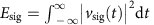, the total energy in the unconverted signal.Figure 3: Temporal and spectral mode conversion of a microwave signal.

To perform mode conversion, we set Vdc≈10 V so the microwave circuit is centred near 7.08 GHz, and send in the signal with the decaying exponential envelope. We apply a microwave pump with a time-dependent amplitude as shown in Fig. 2a to alter Γ(t). The pump amplitude is carefully chosen26 so that we capture almost all of the signal and store it as a vibration of the drumhead (Supplementary Note 3, Supplementary Fig. 4). Only a small amount (4%) of the signal energy is reflected out of the converter, as demonstrated in Fig. 3b. We then set Vdc=0 so that the microwave circuit is centred near 7.34 GHz, and again apply a microwave pump with a time-dependent amplitude as shown in Fig. 2a. The Wigner–Ville distribution of the signal emitted by the mode converter is plotted in Fig. 3b. From the relative energies in the reflected and converted signals, we find the photon number efficiency of the conversion process to be 0.81±0.03, in good agreement with an expected efficiency of (κext/κ)2 × 0.96=0.81±0.04 where 0.96 is the efficiency with which we capture the injected signal26.

The mode converter can be used to change signals between arbitrarily chosen temporal and spectral modes. We demonstrated frequency conversion over approximately 250 MHz; conversion over a larger window is feasible but the mode converter becomes more sensitive to fluctuations in Vdc at higher bias. Although we altered the temporal envelope of a microwave signal from a decaying exponential to a Gaussian, any other envelope is possible, limited only by the bandwidth of the mode converter. The maximum bandwidth is set by the rate at which information can be exchanged between the transmission line and the drumhead, which for this device is limited by the microwave circuit’s bandwidth of κ/2≈2π × 1.3 MHz. The minimum bandwidth is set by the mechanical decoherence rate. Even though energy in the drumhead is lost to the environment at the modest rate of κm=2π × 25 Hz, fluctuations of the environment incoherently drive vibrations of the drumhead. To avoid these environment-driven vibrations, signals must be processed faster than the mechanical decoherence rate of nmκm=2π × 900 Hz, where nm is the thermal equilibrium phonon occupation number of the drumhead (Supplementary Note 4, Supplementary Fig. 5, Supplementary Table 1). Avoiding these excess vibrations is essential when manipulating fragile quantum signals.

To verify that our converter operates quickly and quietly enough to avoid corrupting signals, we collect statistics on single-shot measurements of mode conversion using low-amplitude (10 quanta of energy) signals. We inject a low-amplitude signal with a decaying exponential envelope into the converter, shift its frequency by 90 MHz, and recover a signal with a Gaussian envelope. The central frequency and temporal envelope of the converted signal are already known, and we use this information to perform a least-squares parameter estimation of the quadrature amplitudes X1 and X2 (Supplementary Notes 5 and 6, Supplementary Fig. 6, Supplementary Tables 2 and 3). The quadrature amplitudes are two independent values that can be recast as the amplitude and phase of the converted signal. The result of each single-shot measurement and quadrature amplitude estimation appears as a single point in Fig. 4a. The scatter of the quadrature amplitudes indicates the total amount of noise added to the signal during preparation, mode conversion, and measurement. By comparing the total variance, Var(X1)+Var(X2), of the converted signal with that of measured vacuum noise, we infer the mode converter adds 0.9±0.1 quanta during conversion with a 90 MHz frequency shift, where the uncertainty is the s.e.m. of the inferred added noise for three consecutive data sets, each set formed by running 500 repetitions of the protocol. For mode conversion with frequency shifts 20 MHz, we observe an added noise of 0.5 quanta (Fig. 4c), a value that we entirely attribute to environment-driven vibrations of the drumhead.